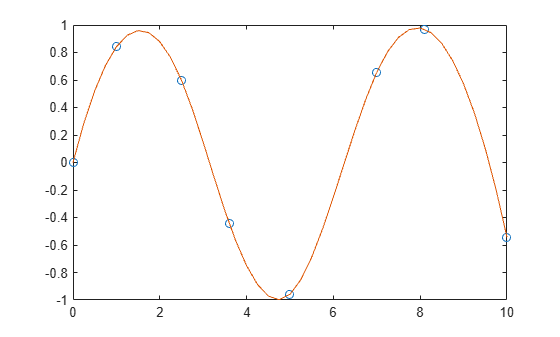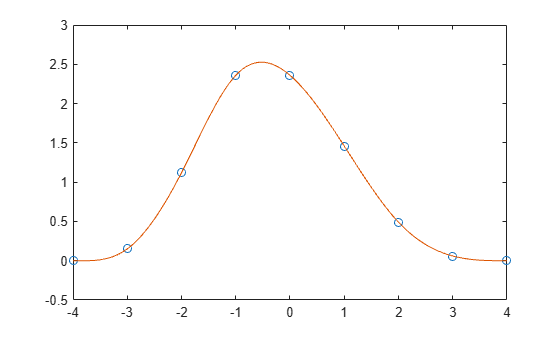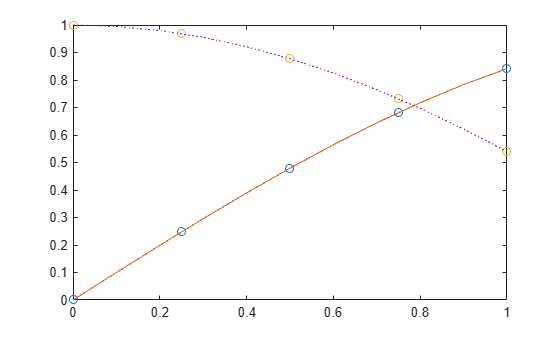# spline

Cubic spline data interpolation

## Syntax

``s = spline(x,y,xq)``
``pp = spline(x,y)``

## Description

example

````s = spline(x,y,xq)` returns a vector of interpolated values `s` corresponding to the query points in `xq`. The values of `s` are determined by cubic spline interpolation of `x` and `y`.```

example

````pp = spline(x,y)` returns a piecewise polynomial structure for use by `ppval` and the spline utility `unmkpp`.```

## Examples

collapse all

Use `spline` to interpolate a sine curve over unevenly-spaced sample points.

```x = [0 1 2.5 3.6 5 7 8.1 10]; y = sin(x); xx = 0:.25:10; yy = spline(x,y,xx); plot(x,y,'o',xx,yy)```Use clamped or complete spline interpolation when endpoint slopes are known. To do this, you can specify the values vector $\mathit{y}$ with two extra elements, one at the beginning and one at the end, to define the endpoint slopes.

Create a vector of data $\mathit{y}$ and another vector with the $\mathit{x}$-coordinates of the data.

```x = -4:4; y = [0 .15 1.12 2.36 2.36 1.46 .49 .06 0];```

Interpolate the data using `spline` and plot the results. Specify the second input with two extra values `[0 y 0]` to signify that the endpoint slopes are both zero. Use `ppval` to evaluate the spline fit over 101 points in the interpolation interval.

```cs = spline(x,[0 y 0]); xx = linspace(-4,4,101); plot(x,y,'o',xx,ppval(cs,xx),'-');```Extrapolate a data set to predict population growth.

Create two vectors to represent the census years from 1900 to 1990 (`t`) and the corresponding United States population in millions of people (`p`).

```t = 1900:10:1990; p = [ 75.995 91.972 105.711 123.203 131.669 ... 150.697 179.323 203.212 226.505 249.633 ];```

Extrapolate and predict the population in the year 2000 using a cubic spline.

`spline(t,p,2000)`
```ans = 270.6060 ```

Generate the plot of a circle, with the five data points `y(:,2),...,y(:,6)` marked with o's. The matrix `y` contains two more columns than does `x`. Therefore, `spline` uses `y(:,1)` and `y(:,end)` as the endslopes. The circle starts and ends at the point (1,0), so that point is plotted twice.

```x = pi*[0:.5:2]; y = [0 1 0 -1 0 1 0; 1 0 1 0 -1 0 1]; pp = spline(x,y); yy = ppval(pp, linspace(0,2*pi,101)); plot(yy(1,:),yy(2,:),'-b',y(1,2:5),y(2,2:5),'or') axis equal```Use spline to sample a function over a finer mesh.

Generate sine and cosine curves for a few values between 0 and 1. Use spline interpolation to sample the functions over a finer mesh.

```x = 0:.25:1; Y = [sin(x); cos(x)]; xx = 0:.1:1; YY = spline(x,Y,xx); plot(x,Y(1,:),'o',xx,YY(1,:),'-') hold on plot(x,Y(2,:),'o',xx,YY(2,:),':') hold off```Compare the interpolation results produced by `spline`, `pchip`, and `makima` for two different data sets. These functions all perform different forms of piecewise cubic Hermite interpolation. Each function differs in how it computes the slopes of the interpolant, leading to different behaviors when the underlying data has flat areas or undulations.

Compare the interpolation results on sample data that connects flat regions. Create vectors of `x` values, function values at those points `y`, and query points `xq`. Compute interpolations at the query points using `spline`, `pchip`, and `makima`. Plot the interpolated function values at the query points for comparison.

```x = -3:3; y = [-1 -1 -1 0 1 1 1]; xq1 = -3:.01:3; p = pchip(x,y,xq1); s = spline(x,y,xq1); m = makima(x,y,xq1); plot(x,y,'o',xq1,p,'-',xq1,s,'-.',xq1,m,'--') legend('Sample Points','pchip','spline','makima','Location','SouthEast')```In this case, `pchip` and `makima` have similar behavior in that they avoid overshoots and can accurately connect the flat regions.

Perform a second comparison using an oscillatory sample function.

```x = 0:15; y = besselj(1,x); xq2 = 0:0.01:15; p = pchip(x,y,xq2); s = spline(x,y,xq2); m = makima(x,y,xq2); plot(x,y,'o',xq2,p,'-',xq2,s,'-.',xq2,m,'--') legend('Sample Points','pchip','spline','makima')```When the underlying function is oscillatory, `spline` and `makima` capture the movement between points better than `pchip`, which is aggressively flattened near local extrema.

## Input Arguments

collapse all

x-coordinates, specified as a vector. The vector `x` specifies the points at which the data `y` is given. The elements of `x` must be unique.

Data Types: `single` | `double`

Function values at x-coordinates, specified as a numeric vector, matrix, or array. `x` and `y` typically have the same length, but `y` also can have exactly two more elements than `x` to specify endslopes.

If `y` is a matrix or array, then the values in the last dimension, `y(:,...,:,j)`, are taken as the values to match with `x`. In that case, the last dimension of `y` must be the same length as `x` or have exactly two more elements.

The endslopes of the cubic spline follow these rules:

• If `x` and `y` are vectors of the same size, then the not-a-knot end conditions are used.

• If `x` or `y` is a scalar, then it is expanded to have the same length as the other and the not-a-knot end conditions are used.

• If `y` is a vector that contains two more values than `x` has entries, then `spline` uses the first and last values in `y` as the endslopes for the cubic spline. For example, if `y` is a vector, then:

• `y(2:end-1)` gives the function values at each point in `x`

• `y(1)` gives the slope at the beginning of the interval located at `min(x)`

• `y(end)` gives the slope at the end of the interval located at `max(x)`

• Similarly, if `y` is a matrix or an `N`-dimensional array with `size(y,N)` equal to `length(x)+2`, then:

• `y(:,...,:,j+1)` gives the function values at each point in `x` for ```j = 1:length(x)```

• `y(:,:,...:,1)` gives the slopes at the beginning of the intervals located at `min(x)`

• `y(:,:,...:,end)` gives the slopes at the end of the intervals located at `max(x)`

Data Types: `single` | `double`

Query points, specified as a scalar, vector, matrix, or array. The points specified in `xq` are the x-coordinates for the interpolated function values `yq` computed by `spline`.

Data Types: `single` | `double`

## Output Arguments

collapse all

Interpolated values at query points, returned as a scalar, vector, matrix, or array.

The size of `s` is related to the sizes of `y` and `xq`:

• If `y` is a vector, then `s` has the same size as `xq`.

• If `y` is an array of size `Ny = size(y)`, then these conditions apply:

• If `xq` is a scalar or vector, then `size(s)` returns `[Ny(1:end-1) length(xq)]`.

• If `xq` is an array, then `size(s)` returns `[Ny(1:end-1) size(xq)]`.

Piecewise polynomial, returned as a structure. Use this structure with the `ppval` function to evaluate the piecewise polynomial at one or more query points. The structure has these fields.

FieldDescription
`form`

`'pp'` for piecewise polynomial

`breaks`

Vector of length `L+1`  with strictly increasing elements that represent the start and end of each of` L` intervals

`coefs`

`L`-by-`k`  matrix with each row `coefs(i,:)` containing the local coefficients of an order `k` polynomial on the `i`th interval, `[breaks(i),breaks(i+1)]`

`pieces`

Number of pieces, `L`

`order`

Order of the polynomials

`dim`

Dimensionality of target

Since the polynomial coefficients in `coefs` are local coefficients for each interval, you must subtract the lower endpoint of the corresponding knot interval to use the coefficients in a conventional polynomial equation. In other words, for the coefficients `[a,b,c,d]` on the interval `[x1,x2]`, the corresponding polynomial is

`$f\left(x\right)=a{\left(x-{x}_{1}\right)}^{3}+b{\left(x-{x}_{1}\right)}^{2}+c\left(x-{x}_{1}\right)+d\text{\hspace{0.17em}}.$`

## Tips

• You also can perform spline interpolation using the `interp1` function with the command `interp1(x,y,xq,'spline')`. While `spline` performs interpolation on rows of an input matrix, `interp1` performs interpolation on columns of an input matrix.

## Algorithms

A tridiagonal linear system (possibly with several right-hand sides) is solved for the information needed to describe the coefficients of the various cubic polynomials that make up the interpolating spline. `spline` uses the functions `ppval`, `mkpp`, and `unmkpp`. These routines form a small suite of functions for working with piecewise polynomials. For access to more advanced features, see `interp1` or the Curve Fitting Toolbox™ spline functions.

 de Boor, Carl. A Practical Guide to Splines. Springer-Verlag, New York: 1978.